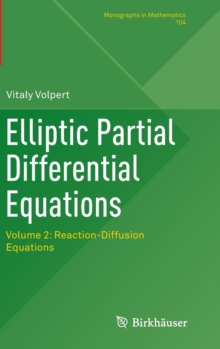# Elliptic Partial Differential Equations : Volume 2: Reaction-Diffusion Equations Hardback

## Part of the Monographs in Mathematics series

#### Description

If we had to formulate in one sentence what this book is about, it might be "How partial differential equations can help to understand heat explosion, tumor growth or evolution of biological species".

These and many other applications are described by reaction-diffusion equations.

The theory of reaction-diffusion equations appeared in the first half of the last century.

In the present time, it is widely used in population dynamics, chemical physics, biomedical modelling.

The purpose of this book is to present the mathematical theory of reaction-diffusion equations in the context of their numerous applications.

We will go from the general mathematical theory to specific equations and then to their applications.

Existence, stability and bifurcations of solutions will be studied for bounded domains and in the case of travelling waves.

The classical theory of reaction-diffusion equations and new topics such as nonlocal equations and multi-scale models in biology will be considered.

#### Information

• Format: Hardback
• Pages: 784 pages, 17 Illustrations, color; 27 Illustrations, black and white; XVIII, 784 p. 44 illus., 17 i
• Publisher: Springer Basel
• Publication Date:
• Category: Differential calculus & equations
• ISBN: 9783034808125

£139.99

£120.75

on all orders

###### Pick up orders

from local bookshops

£128.00

£99.99

£81.45

£99.00

£80.75

£99.99

£81.45

£126.00

£108.95

£109.99

£109.50

£93.08